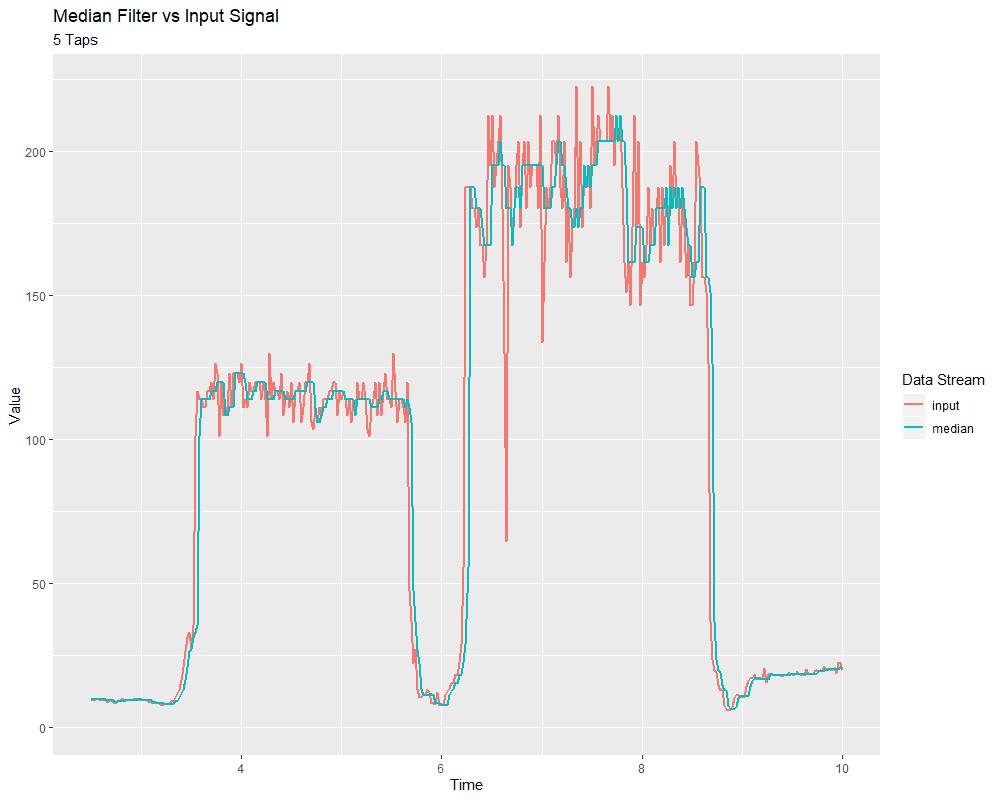# Median Filter¶A robust alternative to the moving-average filter is the median filter. Where a moving average filter takes the arithmetic mean of the input over a moving sample window, a median filter (per the name) takes a median instead.

The median filter is most-useful for removing occasional outliers from an input stream. This makes it particularly well-suited to filtering inputs from distance sensors, which are prone to occasional interference. Unlike a moving average, the median filter will remain completely unaffected by small numbers of outliers, no matter how extreme.

The median filter is supported in WPILib through the `MedianFilter` class (Java, C++).

## Creating a MedianFilter¶

Note

The C++ `MedianFilter` class is templated on the data type used for the input.

Note

Because filters have “memory”, each input stream requires its own filter object. Do not attempt to use the same filter object for multiple input streams.

Creating a `MedianFilter` is simple:

```// Creates a MedianFilter with a window size of 5 samples
MedianFilter filter = new MedianFilter(5);
```
```// Creates a MedianFilter with a window size of 5 samples
frc::MedianFilter<double> filter(5);
```

## Using a MedianFilter¶

Once your filter has been created, using it is easy - simply call the `calculate()` method with the most recent input to obtain the filtered output:

```// Calculates the next value of the output
filter.calculate(input);
```
```// Calculates the next value of the output
filter.Calculate(input);
```